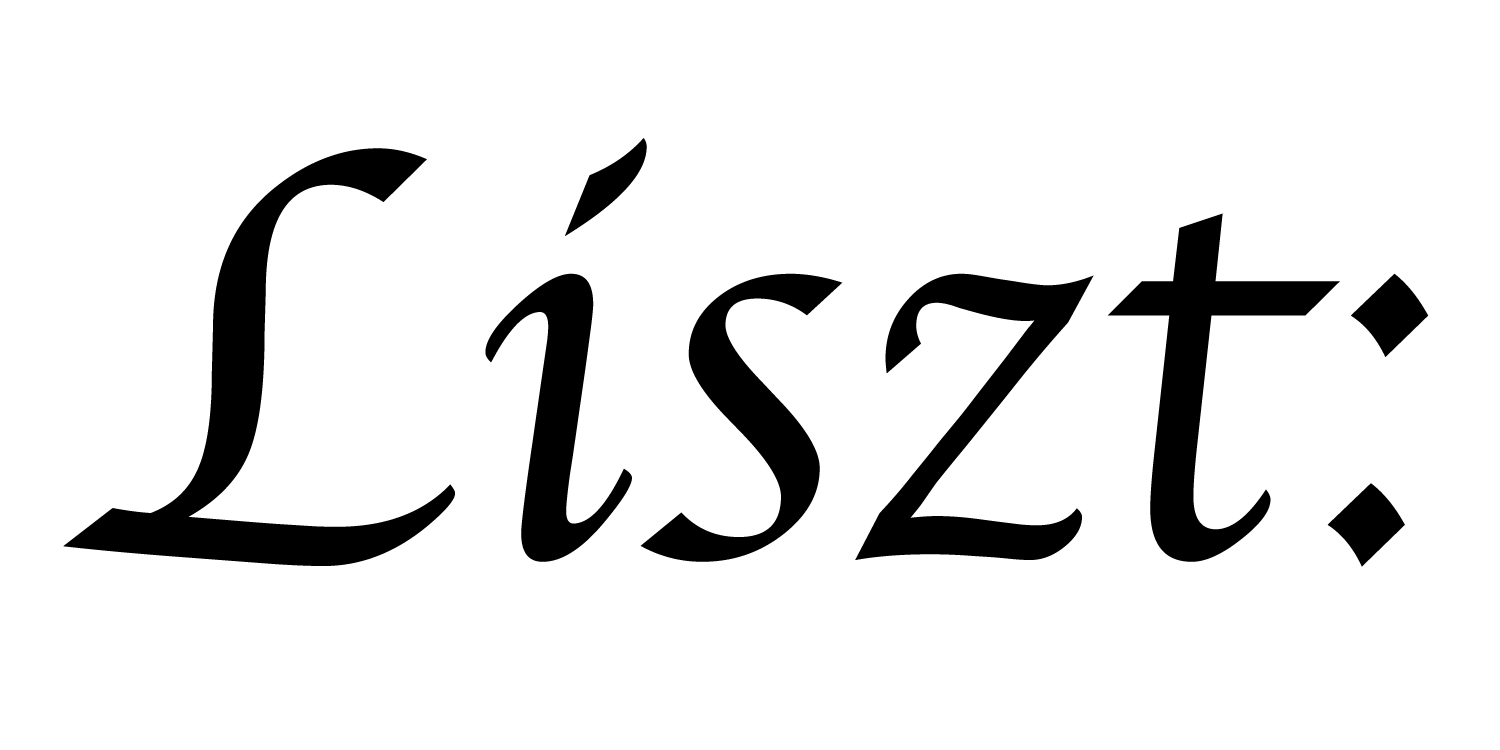# 06: Phases, Reads, Writes, Reductions

In example 05, why did we have to break the heat diffusion step into two functions? Let’s look at some alternate ways we could have written that, and which ways won’t work.

``````import 'ebb'
local L = require 'ebblib'

local ioOff = require 'ebb.domains.ioOff'
local PN    = require 'ebb.lib.pathname'
local mesh  = ioOff.LoadTrimesh( PN.scriptdir() .. 'bunny.off' )

local vdb   = require('ebb.lib.vdb')

local timestep    = L.Constant(L.double, 0.45)
local conduction  = L.Constant(L.double, 1.0)

local function init_temperature(idx)
if idx == 0 then return 1000 else return 0 end
end

local ebb visualize ( v : mesh.vertices )
vdb.color({ 0.5 * v.t + 0.5, 0.5-v.t, 0.5-v.t })
vdb.point(v.pos)
end
``````

We start the program the same way as before, though we define some extra fields for convenience.

``````local ebb compute_update_fail ( v : mesh.vertices )
var sum_t : L.double = 0.0
var count            = 0

for e in v.edges do
count += 1
end

var avg_t  = sum_t / count
var diff_t = avg_t - v.t
v.t += timestep * conduction * diff_t
end
``````

Here’s an obvious way we might try to write the diffusion update. However, if we try to run the simulation using this function, we’ll get a phase-checking error. One of the key features of Ebb is that it only lets programmers write programs that will efficiently parallelize on different architectures. Phase-checking ensures that any kernel execution—i.e. any time a function is executed for all elements—can be run in parallel.

If we try to both read and write the temperature field at the same time, our program will contain data races. Specifically, we’ll get the error `REDUCE(+) Phase is incompatible with READ Phase`, indicating that we cannot reduce and read the `vertices.t` field at the same time. This is why we broke the computation into two functions.

``````local ebb compute_update_swap ( v : mesh.vertices )
var sum_t : L.double = 0.0
var count            = 0

for e in v.edges do
count += 1
end

var avg_t  = sum_t / count
var diff_t = avg_t - v.t
v.new_t = v.t + timestep * conduction * diff_t
end
``````

Rather than storing an update, we could have written the result into a second buffer. Then we can swap the two buffers between each simulation step. This will phase-check safely, but is a different computation for computing the same result.

``````mesh.vertices:NewField('degree', L.double):Load(0)
local ebb compute_degree ( v : mesh.vertices )
for e in v.edges do
v.degree += 1
end
end
mesh.vertices:foreach(compute_degree)

local ebb compute_update_edges ( e : mesh.edges )
var diff_t    = e.head.t - e.tail.t
var d_t       = timestep * conduction * (diff_t / e.tail.degree)
e.tail.new_t += d_t
end
``````

Another possibile variation is that we could write the computation as a per-edge, rather than a per-vertex computation, though now we need to copy rather than swap the data

``````local max_diff = L.Global(L.double, 0)
local ebb measure_max_diff( e : mesh.edges )
var diff_t    = e.head.t - e.tail.t
max_diff max= L.fabs(diff_t)
end
``````

In addition to reductions on fields, we can also reduce values into global variables, which is useful for taking measurements of our simulation. For instance, here we measure the maximum temperature gradient in the mesh. We can then periodically display this information to the user so that they can keep track of the simulation even if they don’t want to watch a live spatial visualization with VDB.

``````for i=1,360 do
--mesh.vertices:foreach(compute_update_fail)

-- do one step with the vertex and swap method
mesh.vertices:foreach(compute_update_swap)
mesh.vertices:Swap('t', 'new_t')

-- and one step with the edge swap-and-zero method
mesh.vertices:Copy { from='t', to='new_t' }
mesh.edges:foreach(compute_update_edges)
mesh.vertices:Swap('t', 'new_t')

vdb.vbegin()
vdb.frame()
mesh.vertices:foreach(visualize)
vdb.vend()

if i % 10 == 0 then -- measure statistics every 10 steps
max_diff:set(0)
mesh.edges:foreach(measure_max_diff)
print( 'iteration #'..tostring(i), 'max gradient: ', max_diff:get() )
end
end
``````

Here we’ve modified the simulation loop to demonstrate the different ways we can compute the diffusion step. If you uncomment the first line, you’ll get the errors discussed above. More detailed descriptions of what phase-checking allows can be found in the full Ebb documentation. In practice, you can assume that phase-checking will fail whenever you have a potential data race.

We’ve also added a bit of code to manage the measurement and reporting of simulation statistics. We can `set()` and `get()` the global’s values from Lua code here before and after the reduction.

a part of the Liszt project and PSAAP II center at Stanford University